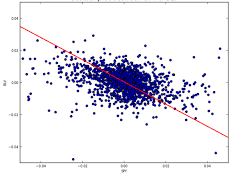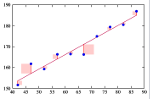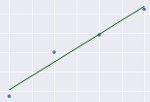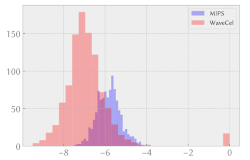# Linear regression analysis Predicting future performance and explaining observations

## OverviewLinear regression analysis means “fitting a straight line to data”. It’s a widely used technique to help model and understand real-world phenomena, which is easy to use and to understand intuitively. It allows prediction of future outputs from the phenomenon you are modelling. Learn how to use plots for exploratory data analysis, to determine whether a linear model might be suitable for your data. Learn how to build univariate and multivariate linear models using the Python statsmodel library.

We also present a number of possible pitfalls when using linear regression, including sample size issues, treatment of outliers and order of effect problems.

This submodule is a part of the risk analysis module.

## Course materialRegression analysis using PythonLecture slides (PDF)Python notebook on regression analysis of health impact of smokingDownload Python notebookRun notebook in MyBinderRun notebook in Google ColabPython notebook on regression analysis of a combined cycle power plantDownload Python notebookRun notebook in MyBinderRun notebook in Google ColabPython notebook with bootstrap regression of helmet performance dataDownload Python notebookRun notebook in MyBinderRun notebook in Google ColabIn these course materials, applications are presented using the NumPy, SciPy and statsmodels libraries for the Python programming language. We have some material on getting started with Python that explains how to install Python on your computer or try out our computational notebooks using free online services.